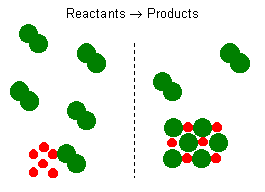# Excess and Limiting Reagents

Key Terms

• Stoichiometric mixture
• Excess reagent
• Limiting reagent

Skills to Develop

• Use stoichiometric calculation to determine excess and limiting reagents in a chemical reaction and explain why.
• Calculate theoretical yields of products formed in reactions that involve limiting reagents.

Chemical reaction equations give the ideal stoichiometric relationship among reactants and products. However, the reactants for a reaction in an experiment are not necessarily a stoichiometric mixture. In a chemical reaction, reactants that are not used up when the reaction is finished are called excess reagents. The reagent that is completely used up or reacted is called the limiting reagent, because its quantity limits the amount of products formed.Let us consider the reaction between solid sodium and chlorine gas. The reaction can be represented by the equation:

$\mathrm{2 Na_{\large{(s)}} + Cl_{2\large{(g)}} \rightarrow 2 NaCl_{\large{(s)}}}$

It represents a reaction of a metal and a diatomic gas chlorine. This balanced reaction equation indicates that two $$\ce{Na}$$ atoms would react with two $$\ce{Cl}$$ atoms or one $$\ce{Cl2}$$ molecule. Thus, if you have 6 $$\ce{Na}$$ atoms, 3 $$\ce{Cl2}$$ molecules will be required. If there is an excess number of $$\ce{Cl2}$$ molecules, they will remain unreacted. We can also state that 6 moles of sodium will require 3 moles of $$\ce{Cl2}$$ gas. If there are more than 3 moles of $$\ce{Cl2}$$ gas, some will remain as an excess reagent, and the sodium is a limiting reagent. It limits the amount of the product that can be formed.

Chemical reactions with stoichiometric amounts of reactants have no limiting or excess reagents.

Example $$\PageIndex{1}$$

Calculate the number of moles of $$\ce{CO2}$$ formed in the combustion of ethane $$\ce{C2H6}$$ in a process when 35.0 mol of $$\ce{O2}$$ is consumed.

HINT

The reaction is

$\ce{2 C2H6 + 7 O2 \rightarrow 4 CO2 + 6 H2O}$

$\mathrm{35.0\: mol\: O_2 \times\dfrac{4\: mol\: CO_2}{7\: mol\: O_2} = 20.0\: mol\: CO_2}$

DISCUSSION

A balanced equation for the reaction is a basic requirement for identifying the limiting reagent even if amounts of reactants are known.

Example $$\PageIndex{2}$$

Two moles of $$\ce{Mg}$$ and five moles of $$\ce{O2}$$ are placed in a reaction vessel, and then the $$\ce{Mg}$$ is ignited according to the reaction

$$\mathrm{Mg + O_2 \rightarrow MgO}$$.

Identify the limiting reagent in this experiment.

HINT

Before a limiting reagent is identified, the reaction must be balanced. The balanced reaction is

$\mathrm{2 Mg + O_2 \rightarrow 2 MgO}$

Thus, two moles of $$\ce{Mg}$$ require only ONE mole of $$\ce{O2}$$. Four moles of oxygen will remain unreacted. Therefore, oxygen is the excess reagent, and $$\ce{Mg}$$ is the limiting reagent.

DISCUSSION

How many moles of $$\ce{MgO}$$ is formed?
What is the weight of $$\ce{MgO}$$ formed?

## Skill Developing Problems

1. Which of the following will react most vigorously with $$\ce{Cl2}$$?

$$\ce{Li}$$, $$\ce{Na}$$, $$\ce{K}$$, $$\ce{Fe}$$, $$\ce{Al}$$, $$\ce{Mg}$$, or $$\ce{Ar}$$?

Hint: Potassium, $$\ce{K}$$

Skill -
Predict reactivity from location of element in the periodic table.

1. One mole of $$\ce{Cl2}$$ molecules would react with how many moles of $$\ce{Na}$$ metal?

Hint: Two moles

Skill -
Write a reaction equation for a chemical reaction.

1. At room temperature (25 °C) what is the state of sodium: solid, gas or liquid?

Hint: Solid

Skill -
Know where to look up properties of elements or substances.

1. Which of the following is the best conductor: sodium metal, solid $$\ce{NaCl}$$, chlorine gas, dilute $$\ce{NaCl}$$ solution, or molten $$\ce{NaCl}$$?

Hint: Sodium metal

1. What positive ions are present in solutions of $$\ce{NaCl}$$?

Hint: sodium ions

1. If you put equal weights of sodium metal and chlorine gas into a reaction vessel, which is the limiting reagent?

Hint: chlorine is the limiting reagent

Skill -
Identify excess and limiting reagent.

1. Equal weights of $$\ce{H2}$$ and $$\ce{O2}$$ are placed in a balloon and then ignited. Assuming reaction goes to completion, which gas is the excess reagent?

Hint: hydrogen

1. Equal weights of $$\ce{H2}$$ and $$\ce{F2}$$ are placed in a balloon and then ignited. Assuming reaction goes to completion, which gas is the limiting reagent?

Hint: Fluorine

Skill - Identify the excess and limiting reagent.

2. The reaction:
$$\mathrm{2 Al_{\large{(s)}} + Fe_2O_3_{\large{(s)}} \rightarrow 2 Fe_{\large{(liquid)}} + Al_2O_3}$$
takes place in the thermite mixture when it is ignited by a magnesium ribbon. A thermite mixture contains a mass ratio of 1 to 2 for $$\ce{Al}$$ and $$\ce{Fe2O3}$$. Which one is the limiting reagent?

Hint: Iron oxide

Discussion -
A stoichiometric mixture has a mass ratio of 54:160 (nearly 1:3) for $$\ce{Al:Fe2O3}$$.